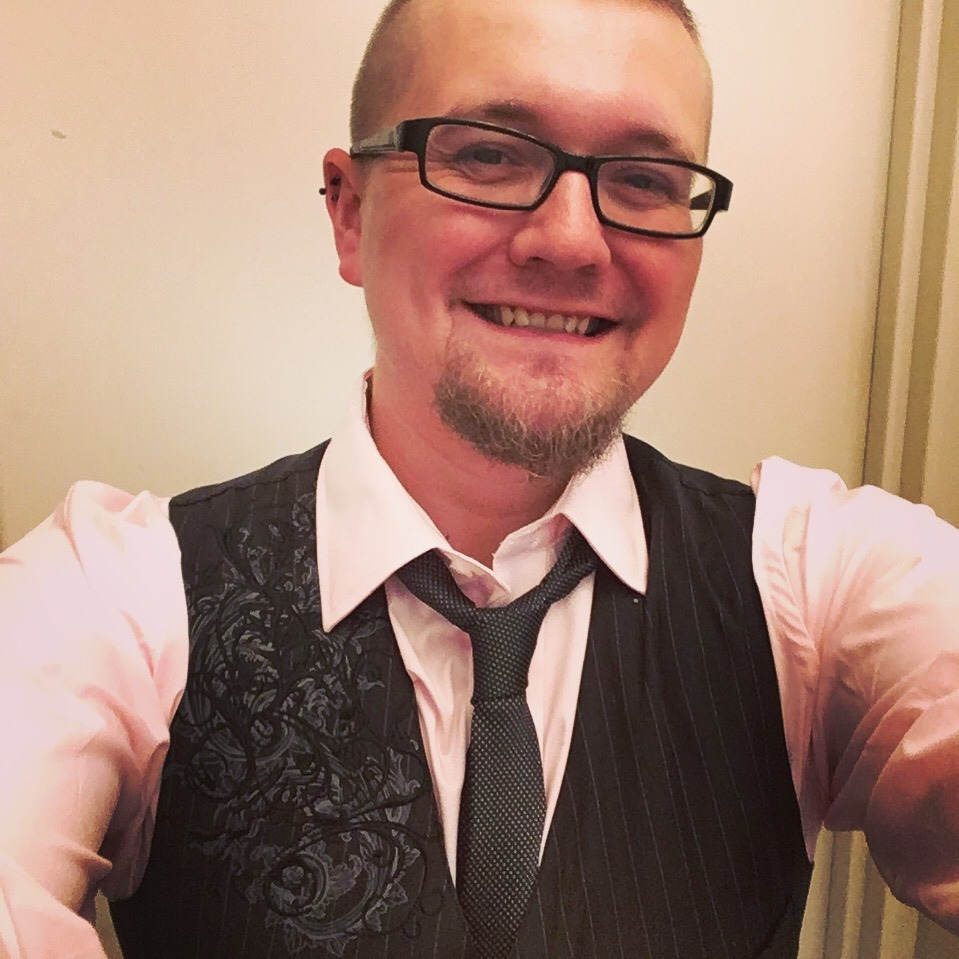# The Great Conversation: Mathematics## Few disciplines are as practical as mathematics—or as often pursued out of sheer love of knowledge.

Of the Seven Liberal Arts codified by the ancients, two (geometry and arithmetic) are mathematical disciplines, while three more (dialectic, astronomy, and music) require mathematical knowledge or can be analyzed in a mathematical form. It is a common refrain, and probably has been for centuries, from the teacher or parent to the reluctant student that every adult profession involves math, so yes, you do have to finish your homework. But one interesting facet of this discipline is how many of the advances made it in, which so often proved highly useful later, were motivated by curiosity of the most un-practical kind.

The earliest mathematics of Babylon, Egypt, India, and China do appear to have been driven by practical requirements, specifically those of astronomy (which governed the calendar, in turn a necessity of all agriculture) and architecture. Pythagoras, he of the theorem, took a quite different approach to the subject: he considered number to be the defining force of the whole universe, and understood mathematics as a mystical discipline. He discovered ways of understanding musical intervals as numerical proportionsfor instance, on a lyre, a string that is half as long as another string will sound a note one octave higher. It was the Pythagorean community that proved the existence of irrational numbers, i.e., numbers like π that cannot be expressed as the ratio of two integers. (Unfortunately for the Pythagorean who made this discovery, the community was outraged by this discovery, which they saw as a fundamental challenge to their belief in numberso much so that, according to one account, they flung him off a ship he was traveling in!)

Later mathematicians of antiquity saw this as expressing the difference between number and magnitude. Arithmetic was considered the study of the former, and geometry of the latter: numbers were discrete values (e.g., jumping from 4 to 5), measuring things that boil down to irreducible units, while magnitudes were continuous, measuring things like angles, volumes, and times that are infinitely divisible. Geometers abound in this period; Archimedes comes to mind, who claimed to have calculated the volume of the cosmos precisely enough to know how many grains of sand it would take to fill it. Arguably the greatest was Euclid, a scholar at the famous school of Alexandria, whose compendium The Elements remained the standard textbook on the subject for twenty-two centuries.

In the Middle Ages, Arabian, Persian, and Indian mathematicians  expanded the field of algebra (so much so that its name comes from the Arabic al-jabr, “the restoring of broken parts”). Mathematics as studied in Europe received a shot in the arm from them in the thirteenth century, when Arabic numerals and decimal notation were introduced for use by Leonardo Fibonacci. The more cumbersome Roman system, though it lingers to this day for some uses, was ultimately replaced; the far greater concision of the Arabic system enabled calculations that were even simpler and faster than the abacus had allowed.

The seventeenth century saw the discovery of the techniques of calculustwice. Unbeknownst to each other, both Sir Isaac Newton and Gottfried Leibniz deduced these principles, which mathematically describe change, the slopes of curves, and (by extension) a number of fundamental concepts in the sciences, such as acceleration in physics.

Advances in calculation techniques and number theory continue to this day. In a lecture in 1900, twenty-three unsolved problems and conjectures had been laid before the International Congress of Mathematicians, and much of the twentieth century was spent in solving them, or in proving definitively that they could not be solved. Fermat’s Last Theorem, a conjecture of a seventeenth-century French mathematician, was tackled by English mathematician Andrew Wiles. He worked on it secretly for six years, andafter a false start that almost dashed his hopesWiles finally proved Fermat’s Last Theorem in 1995, opening vast new fields of study and demonstrating the utility of new tools of proof. And he did it for the most human of motives: because he was curious to know what the answer was.